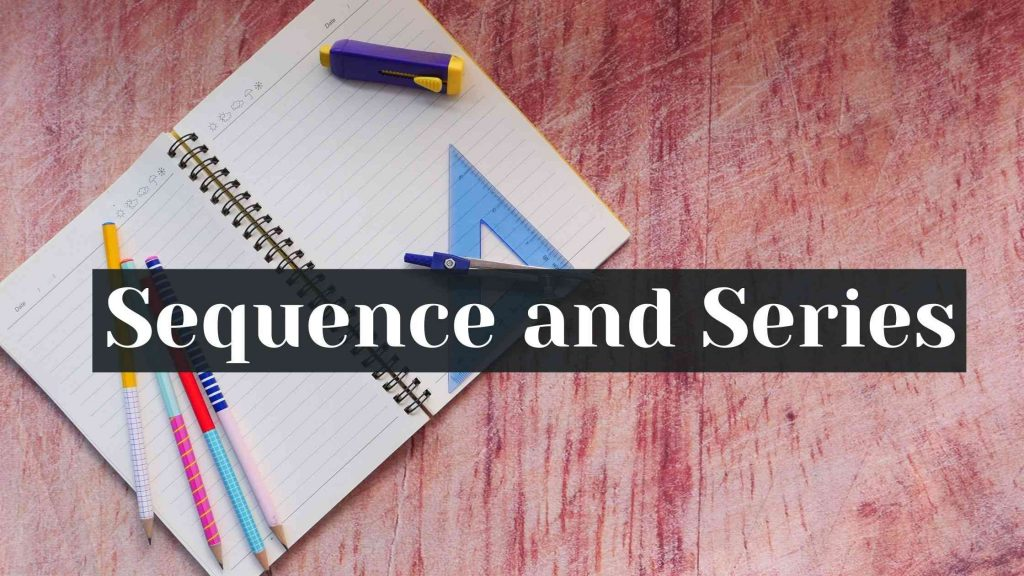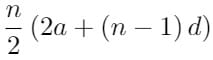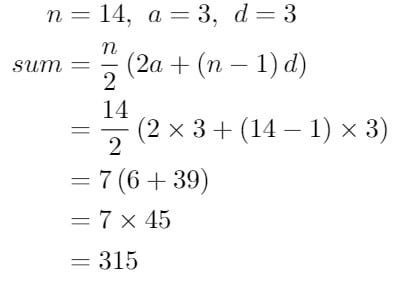Maths
Study Material

# Sequence and Series with solved problems

We see sequences everywhere in our life, from calendars to mobile phones, from problem numbers to house numbers. Anywhere you see you can get hold of a sequence. So, let us spend time to know what exactly is a sequence and few related concepts.

4 minutes long
Posted by Tanwir Silar, 20/7/2021Hesap Oluştur

Got stuck on homework? Get your step-by-step solutions from real tutors in minutes! 24/7. Unlimited.

A sequence is a set of numbers either having a theoretical scheme ( verbal description) or having a general formula for the nth term. A finite sequence is a sequence with finite terms in it whereas, an infinite sequence is a sequence with infinitely many terms. A series is the sum of the terms of a sequence, which has a definite formula for it’s nth term.
Mathematically, if a1, a2, a3, … , an is a sequence, then the series associated with it is: a1 + a2 + a3 + … + an.
A series is finite or infinite according to the sequence it is associated with.

## What is a term of a sequence?

The various numbers of a sequence are called its terms. The nth term is the number at the nth position and is denoted by an. The nth term is also called the general term of sequence.

## Different types of sequences

Depending on the formula for the general term, we have different types of sequences. For example, some Common sequences are:

## Arithmetic Sequences

If any two successive terms of a sequence differ by a constant number, known as common difference, then the sequence is an arithmetic sequence.
For example:
i) 2, 5, 8 ,11, 14, 17
ii) 10, 5, 0, -5, -10, -15
iii) Set of Natural numbers
iv) Set of Whole numbers
v) Set of Even numbers

## Geometric Sequences

If the ratio of any two successive terms of a sequence is constant, then the sequence is a geometric sequence. Here, the ratio of an+1 to an is known as common ratio.
For example:
i) 2, 1, 1/2, 1/4, 1/8.
ii) 3, 6, 12, 24, 48.
iii) 5, 25, 125, 625, 3125.

## Harmonic Sequences

If the inverses of any two successive terms of a sequence is constant, then the sequence is a harmonic sequence. Thus, the inverses of terms of a harmonic sequence form an arithmetic sequence, by definition.
Ex:
i) 1/4, 1/8, 1/12, 1/16, 1/20.
ii) 1, 1/2, 1/3, 1/4, 1/5, 1/6.

## Fibonacci Sequence*

Each term of the sequence is the sum of the two preceding terms, with the first two terms fixed as 0, 1.
For instance, the first 10 terms of a fibonacci sequence are 0, 1, 1, 2 3, 5, 8, 13, 21, 34.

## Solved problems on sequence and series

Example 1: If 3, 5, 7, 9, … ,53 is an arithmetic sequence, then find the common difference and the 21st term in the sequence?

Solution:
Common difference of an Arithmetic sequence is the difference between any two successive terms of the sequence.
Taking the first two terms. a2 = 5, a1 = 3,
Common difference = d = an – an-1
= a2 – a1 = 5 – 3
d = 2.
Formula for the nth term of arithmetic sequence = a1 + (n – 1) d
For n = 21, we have a21 = 3 + (21 – 1) * 2
= 3+ 40 = 43
21st term of the sequence = a21 = 43.

Example 2: Find the sum of the series 3 + 6 + 9 + … + 42.

Solution:
Since the successive terms of the series have a constant difference, the given series is an arithmetic series.
Formula for sum of an arithmetic series =Where,
n = number of terms in the series,
a = first term of the series,
d = common difference of the series.
d = a2 – a1
= 6 – 3
d = 3
Finding n, we know that the nth term is 42
Therefore, 42 = a + (n-1) d
= 3 + (n-1) * 3
39 = (n-1) * 3
n = 1 + 13 = 14.